#SOLVING EXPONENTIAL EQUATIONSTo solve an exponential equation, take the log of both sides, and solve for the variable.Example 1: Solve for x in the equation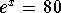.

Solution:

Step 1: Take the natural log of both sides: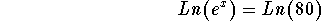Step 2: Simplify the left side of the above equation using Logarithmic Rule 3: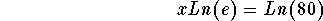Step 3: Simplify the left side of the above equation: Since Ln(e)=1, the equation reads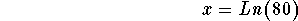Ln(80) is the exact answer and x=4.38202663467 is an approximate answer because we have rounded the value of Ln(80)..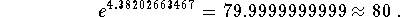Example 2: Solve for x in the equation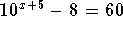Solution:

Step 1: Isolate the exponential term before you take the common log of both sides. Therefore, add 8 to both sides: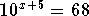Step 2: Take the common log of both sides: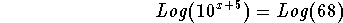Step 3: Simplify the left side of the above equation using Logarithmic Rule 3: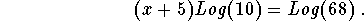Step 4: Simplify the left side of the above equation: Since Log(10) = 1, the above equation can be written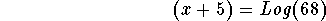Step 5: Subtract 5 from both sides of the above equation: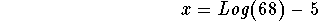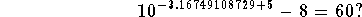Yes it does.

Example 3: Solve for x in the equation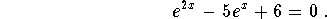Solution:

Step 1: When you graph the left side of the equation, you will note that the graph crosses the x-axis in two places. This means the equation has two real solutions.
Step 2: Rewrite the equation in quadratic form: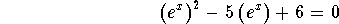Step 3: Factor the left side of the equation:can now be written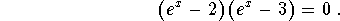Step 4: Solve for x. Note: The product of two terms can only equal zero if one or both of the two terms is zero.
Step 5: Set the first factor equal to zero and solve for x: If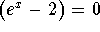, then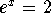and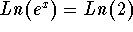and x=Ln(2) is the exact answer or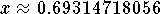is an approximate answer.
Step 6: Set the second factor equal to zero and solve for x: If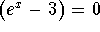, then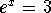and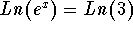and x=Ln(3) is the exact answer or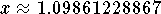is an approximate answer. The exact answers are Ln(3) and Ln(2) and the approximate answers are 0.69314718056 and 1.09861228867.

Check: These two numbers should be the same numbers where the graph crosses the x-axis.

Remark: Why did we choose the Ln in Example 3? Because we know that Ln(e) = 1.

If you would like to review another example, click on Example.

Work the following problems. If you want to review the answer and the solution, click on answer.

Problem 1: Solve for x in the equation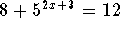.

Problem 2: Solve for x in the equation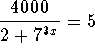.

Problem 3: Solve for x in the equation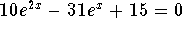.

Problem 4: Solve for x in the equation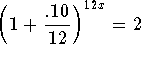.

Problem 5: Solve for x in the equation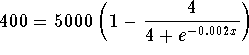.

Problem 6: Solve for x in the equation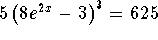.[Menu Back to Exponential Functions] [Go on to Solving Logarithmic Equations]

[Algebra] [Trigonometry] [Complex Variables]S.O.S MATHematics home page

Do you need more help? Please post your question on our S.O.S. Mathematics CyberBoard.Author: Nancy Marcus